For some time now Toad’s SQL Editor has offered several very basic SQL code refactoring capabilities. But to be honest those refactoring features did not see extensive use or adoption as they were so basic. Thus Toad 12.1, releasing Sept 19th 2013, will add several major SQL refactoring enhancements sure to be a huge success. In this blog I’m covering just the top four which I think will be the most useful. Let’s look at some of the clear and cool refactoring “home runs” coming soon to a Toad near you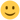NOTE – All the examples below were accomplished via the Toad Editor’s Right-Hand-Mouse (i.e. RHM) menu and selecting Refactor. There are plans to create a new Refactoring toolbar for better exposure, but that’s not available yet in the current beta being used for this demo.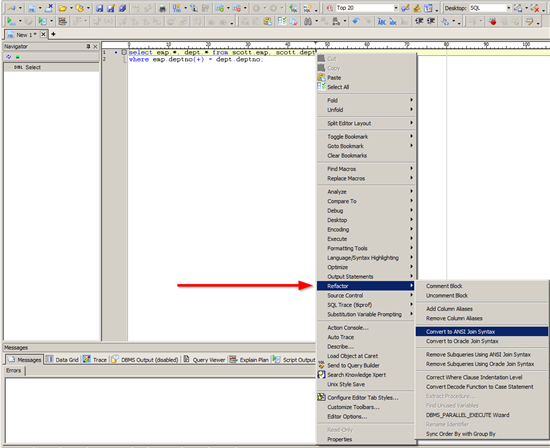Refactor #1 – Convert to/from ANSI Join Syntax

Oracle has stated that going forward we all should be using the ANSI join syntax pretty much exclusively. Yes – I know that early versions had lots of bugs, but we’re many years past that point. However many of us remain more comfortable and quicker with the old syntax, plus we have tons of SQL code already written in the old syntax. Now Toad will make the Join syntax a moot issue – as you can switch back and forth between join syntax styles with the click of a button. Here’s an example of a six-way join converted from Oracle join syntax to ANSI – and it took all of just one second to convert. Remember – Toad offers converting in both directions, so to and from ANSI join syntax.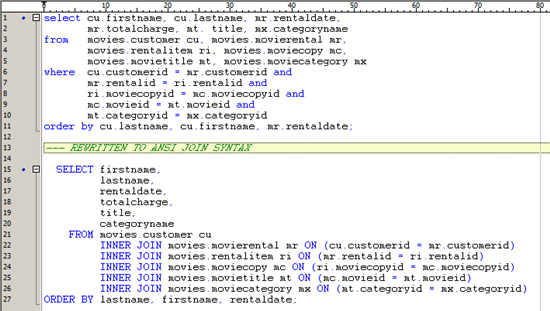Refactor #2 – Remove Sub-Queries Using ANSI/Oracle Join Syntax

For many people reading and comprehending a nested sub-query is not as easy as an equivalent join. In fact in many cases the Oracle optimizer actually makes such conversions without the user ever knowing about it. Now Toad will understanding nested sub-queries a moot issue – as you can now convert to the equivalent join syntax with just the click of a button. Here’s a simple example of two nested sub-queries converted to ANSI join syntax – and it took all of just one second to convert. Remember – Toad offers converting nested sub-queries to both Oracle and ANSI join syntax.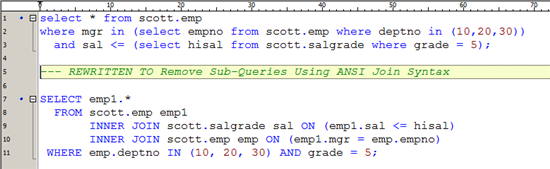Refactor #3 – Correct WHERE Clause Indention Level

This next example is very complex. But it represents real world problems routinely encountered when writing nested sub-queries. Notice that the original SQL is 18 lines with nested sub-queries up to three levels deep. Now note that the rewrite is just 10 lines – a 44% reduction – with sub-queries now only two levels deep. These coding mistakes fall into two categories – cut and paste errors/oversights and nested sub-query overload (i.e. not being able to see the trees from the forest). Let’s examine what Toad saw and corrected.

The lines marked as #1 are simple WHERE clause conditions nested at the wrong level (i.e. too deep). Those lines can be pushed out to the main WHERE clause since they’re only dependent on table “e1”. Furthermore they are duplicates and hence one can be dropped.

The lines marked as #2 are also simple WHERE clause conditions nested at the wrong level (i.e. too deep). Those lines can be pushed out to the second level WHERE clause since they’re only dependent on table “d1”. In this case there are no duplicates to remove.

The lines marked as #3 are WHERE clause sub-queries clauses nested at the wrong level (i.e. too deep). Those lines can be pushed out to the main WHERE clause since they’re only dependent on table “e1”. Furthermore they are duplicates and hence one can be dropped.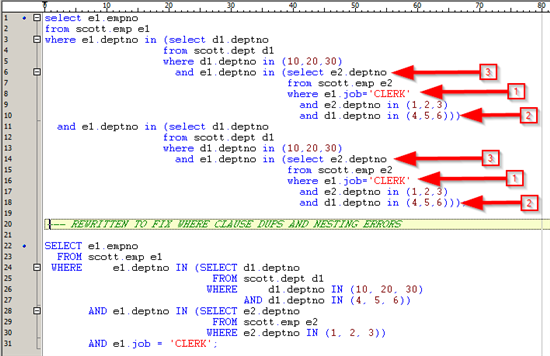Would you have been able to spot and correct this kind of mistakes? Maybe – but it’s both a visual and mental challenge to be able to make such corrections. Now Toad does it auto-magically for you. The end result is SQL code that’s far easier to both read and maintain. Plus it also is far more efficient – look at the before vs. after explain plans shown here. Note – calling SQL Tuning tools such as Toad Xpert’s Auto or Advanced Optimize, or OEM’s Tuning Advisor would not find nor correct such coding mistakes! They operate on the premise of optimizing properly structured SQL – not correcting SQL coding mistakes.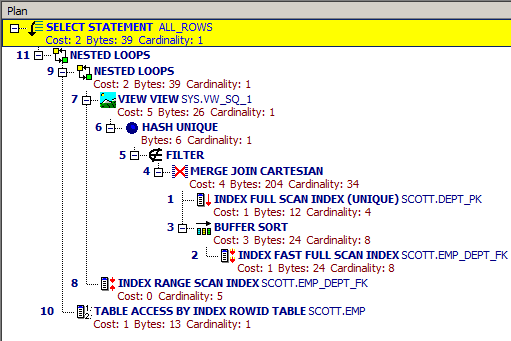Figure 1: Explain Plan Before Refactor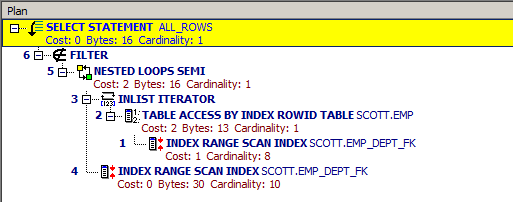Figure 2: Explain Plan After Refactor

Refactor #4 – Convert DECODE Function to CASE Statement

Prior to ASNI SQL defining a CASE statement, Oracle had the DECODE function for handling “if then else” type logic. Sometime after ANSI defined the CASE statement, Oracle then added support for it. However many of us remain more comfortable and quicker using the old DECODE function, plus we have tons of SQL code already written using DECODE. Now Toad will make DECODE vs. CASE syntax a moot issue – as you can now easily convert all DECODE function calls to CASE statements with just the click of a button. No more counting parenthesis levels while trying to make the switch! Here’s a real-world DECODE to CASE statement conversion example from my recent blog on calculating IOPS. This may be a very long example, but it very clearly shows the power of auto-magic correction`SELECT end_time,       ROUND(sr/inttime,3) sri,       ROUND(sw/inttime,3) swi,       ROUND((sr+sw)/inttime,3) tsi,       ROUND(sr/DECODE((sr+sw),0,1,(sr+sw))*100,3)srp,       ROUND(sw/DECODE((sr+sw),0,1,(sr+sw))*100,3) swp,       ROUND(lr/inttime,3) lri,       ROUND(lw/inttime,3) lwi,       ROUND((lr+lw)/inttime,3) tli,       ROUND(lr/DECODE((lr+lw),0,1,(lr+lw))*100,3) lrp,       ROUND(lw/DECODE((lr+lw),0,1,(lr+lw))*100,3) lwp,       ROUND((tbr/inttime)/1048576,3) tr,       ROUND((tbw/inttime)/1048576,3) tw,       ROUND(((tbr+tbw)/inttime)/1048576,3) tmFROM (SELECT beg.snap_id beg_id, end.snap_id end_id,       beg.begin_interval_time, beg.end_interval_time,       end.begin_interval_time begin_time, end.end_interval_time end_time,       (extract(day   from (end.end_interval_time - end.begin_interval_time))*86400)+       (extract(hour   from (end.end_interval_time - end.begin_interval_time))*3600)+       (extract(minute from (end.end_interval_time - end.begin_interval_time))*60)+       (extract(second from (end.end_interval_time - end.begin_interval_time))*01) inttime,       decode(end.startup_time,end.begin_interval_time,end.sr,(end.sr-beg.sr))   sr,       decode(end.startup_time,end.begin_interval_time,end.sw,(end.sw-beg.sw))   sw,       decode(end.startup_time,end.begin_interval_time,end.lr,(end.lr-beg.lr))   lr,       decode(end.startup_time,end.begin_interval_time,end.lw,(end.lw-beg.lw))   lw,       decode(end.startup_time,end.begin_interval_time,end.tbr,(end.tbr-beg.tbr)) tbr,       decode(end.startup_time,end.begin_interval_time,end.tbw,(end.tbw-beg.tbw)) tbwFROM(SELECT dba_hist_snapshot.snap_id, startup_time, begin_interval_time, end_interval_time,sum(decode(stat_name,'physical read total IO requests',value,0)-decode(stat_name,'physical read total multi block requests',value,0)) sr,sum(decode(stat_name,'physical write total IO requests',value,0)-decode(stat_name,'physical write total multi block requests',value,0)) sw,sum(decode(stat_name,'physical read total multi block requests',value,0)) lr,sum(decode(stat_name,'physical write total multi block requests',value,0)) lw,sum(decode(stat_name,'physical read total bytes',value,0)) tbr,sum(decode(stat_name,'physical write total bytes',value,0)) tbw   FROM wrh\$_sysstat, wrh\$_stat_name, dba_hist_snapshotWHERE wrh\$_sysstat.stat_id = wrh\$_stat_name.stat_id   AND wrh\$_sysstat.snap_id = dba_hist_snapshot.snap_idgroup by dba_hist_snapshot.snap_id, startup_time, begin_interval_time, end_interval_time) beg,(SELECT dba_hist_snapshot.snap_id, startup_time, begin_interval_time, end_interval_time,sum(decode(stat_name,'physical read total IO requests',value,0)-decode(stat_name,'physical read total multi block requests',value,0)) sr,sum(decode(stat_name,'physical write total IO requests',value,0)-decode(stat_name,'physical write total multi block requests',value,0)) sw,sum(decode(stat_name,'physical read total multi block requests',value,0)) lr,sum(decode(stat_name,'physical write total multi block requests',value,0)) lw,sum(decode(stat_name,'physical read total bytes',value,0)) tbr,sum(decode(stat_name,'physical write total bytes',value,0)) tbw   FROM wrh\$_sysstat, wrh\$_stat_name, dba_hist_snapshotWHERE wrh\$_sysstat.stat_id = wrh\$_stat_name.stat_id   AND wrh\$_sysstat.snap_id = dba_hist_snapshot.snap_idgroup by dba_hist_snapshot.snap_id, startup_time, begin_interval_time, end_interval_time) endWHERE beg.snap_id + 1 = end.snap_id)order by 1;`

— REWRITTEN TO COVERT DECODE FUNCTION TO CASE STATEMENT

`SELECT end_time,         ROUND (sr / inttime, 3) sri,         ROUND (sw / inttime, 3) swi,         ROUND ( (sr + sw) / inttime, 3) tsi,         ROUND ( sr               / CASE                   WHEN sr + sw = 0 THEN 1                   ELSE (sr + sw)                 END               * 100,               3)           srp,         ROUND ( sw               / CASE                   WHEN sr + sw = 0 THEN 1                   ELSE (sr + sw)                 END               * 100,               3)           swp,         ROUND (lr / inttime, 3) lri,         ROUND (lw / inttime, 3) lwi,         ROUND ( (lr + lw) / inttime, 3) tli,         ROUND ( lr               / CASE                   WHEN lr + lw = 0 THEN 1                   ELSE (lr + lw)                 END               * 100,               3)           lrp,         ROUND ( lw               / CASE                   WHEN lr + lw = 0 THEN 1                   ELSE (lr + lw)                 END               * 100,               3)           lwp,         ROUND ( (tbr / inttime) / 1048576, 3) tr,         ROUND ( (tbw / inttime) / 1048576, 3) tw,         ROUND ( ( (tbr + tbw) / inttime) / 1048576, 3) tm   FROM (SELECT beg.snap_id beg_id,                 end.snap_id end_id,                 beg.begin_interval_time,                 beg.end_interval_time,                 end.begin_interval_time begin_time,                 end.end_interval_time end_time,                   ( EXTRACT (                       DAY FROM ( end.end_interval_time                                 - end.begin_interval_time))                   * 86400)                 + ( EXTRACT (                       HOUR FROM ( end.end_interval_time                                   - end.begin_interval_time))                   * 3600)                 + ( EXTRACT (                       MINUTE FROM ( end.end_interval_time                                     - end.begin_interval_time))                   * 60)                 + ( EXTRACT (                       SECOND FROM ( end.end_interval_time                                     - end.begin_interval_time))                   * 01)                   inttime,                 CASE                   WHEN end.startup_time = end.begin_interval_time THEN end.sr                   ELSE (end.sr - beg.sr)                 END                   sr,                 CASE                   WHEN end.startup_time = end.begin_interval_time THEN end.sw                   ELSE (end.sw - beg.sw)                 END                   sw,                 CASE                   WHEN end.startup_time = end.begin_interval_time THEN end.lr                   ELSE (end.lr - beg.lr)                 END                   lr,                 CASE                   WHEN end.startup_time = end.begin_interval_time THEN end.lw                   ELSE (end.lw - beg.lw)                 END                   lw,                 CASE                   WHEN end.startup_time = end.begin_interval_time THEN end.tbr                   ELSE (end.tbr - beg.tbr)                 END                   tbr,                 CASE                   WHEN end.startup_time = end.begin_interval_time THEN end.tbw                   ELSE (end.tbw - beg.tbw)                 END                   tbw           FROM ( SELECT dba_hist_snapshot.snap_id,                           startup_time,                           begin_interval_time,                           end_interval_time,                          SUM (                               CASE                                 WHEN stat_name =                                       'physical read total IO requests' THEN                                   VALUE                                 ELSE                                   0                               END                             - CASE                                 WHEN stat_name =                                       'physical read total multi block requests' THEN                                   VALUE                                 ELSE                                   0                               END)                             sr,                           SUM (                               CASE                                 WHEN stat_name =                                       'physical write total IO requests' THEN                                   VALUE                                 ELSE                                  0                               END                             - CASE                                 WHEN stat_name =                                       'physical write total multi block requests' THEN                                  VALUE                                 ELSE                                   0                               END)                             sw,                           SUM (                             CASE                               WHEN stat_name =                                     'physical read total multi block requests' THEN                                 VALUE                               ELSE                                 0                             END)                             lr,                           SUM (                             CASE                               WHEN stat_name =                                     'physical write total multi block requests' THEN                                 VALUE                               ELSE                                 0                             END)                             lw,                           SUM (                             CASE                               WHEN stat_name = 'physical read total bytes' THEN                                 VALUE                               ELSE                                 0                             END)                             tbr,                           SUM (                             CASE                               WHEN stat_name = 'physical write total bytes' THEN                                 VALUE                               ELSE                                 0                             END)                             tbw                     FROM wrh\$_sysstat, wrh\$_stat_name, dba_hist_snapshot                     WHERE wrh\$_sysstat.stat_id = wrh\$_stat_name.stat_id                      AND wrh\$_sysstat.snap_id = dba_hist_snapshot.snap_id                 GROUP BY dba_hist_snapshot.snap_id,                           startup_time,                           begin_interval_time,                           end_interval_time) beg,                 ( SELECT dba_hist_snapshot.snap_id,                           startup_time,                           begin_interval_time,                           end_interval_time,                           SUM (                               CASE                                 WHEN stat_name =                                       'physical read total IO requests' THEN                                   VALUE                                 ELSE                                  0                               END                             - CASE                                 WHEN stat_name =                                       'physical read total multi block requests' THEN                                   VALUE                                 ELSE                                   0                               END)                             sr,                           SUM (                               CASE                               WHEN stat_name =                                       'physical write total IO requests' THEN                                   VALUE                                 ELSE                                   0                               END                             - CASE                                 WHEN stat_name =                                       'physical write total multi block requests' THEN                                   VALUE                                ELSE                                   0                               END)                             sw,                           SUM (                             CASE                               WHEN stat_name =                                   'physical read total multi block requests' THEN                                 VALUE                               ELSE                                 0                             END)                             lr,                           SUM (                             CASE                               WHEN stat_name =                                     'physical write total multi block requests' THEN                                 VALUE                               ELSE                                 0                             END)                             lw,                           SUM (                             CASE                              WHEN stat_name = 'physical read total bytes' THEN                                 VALUE                               ELSE                                 0                             END)                             tbr,                          SUM (                             CASE                               WHEN stat_name = 'physical write total bytes' THEN                                 VALUE                               ELSE                                 0                            END)                             tbw                     FROM wrh\$_sysstat, wrh\$_stat_name, dba_hist_snapshot                     WHERE wrh\$_sysstat.stat_id = wrh\$_stat_name.stat_id                       AND wrh\$_sysstat.snap_id = dba_hist_snapshot.snap_id                 GROUP BY dba_hist_snapshot.snap_id,                           startup_time,                           begin_interval_time,                           end_interval_time) end           WHERE beg.snap_id + 1 = end.snap_id)ORDER BY 1;`
Bert Scalzo is a guest-blogger for Quest and a renowned database expert, Oracle® ACE, author, database technology consultant, and formerly a member of Dell Software’s TOAD dev team. With three decades of Oracle® database experience to draw on, Bert’s webcasts garner high attendance and participation rates. His work history includes time at both Oracle Education and Oracle Consulting. Bert holds several Oracle Masters certifications and has an extensive academic background that includes a BS, MS and Ph.D. in computer science, as well as an MBA, and insurance industry designations. Bert is a highly sought-after speaker who has presented at numerous Oracle conferences and user groups, including OOW, ODTUG, IOUG, OAUG, RMOUG and many others. Bert enjoys sharing his vast knowledge on data modeling, database benchmarking, database tuning and optimization, "star schema" data warehouses, Linux® and VMware®. As a prolific writer, Bert has produced educational articles, papers and blogs for such well-respected publications as the Oracle Technology Network (OTN), Oracle Magazine, Oracle Informant, PC Week (eWeek), Dell Power Solutions Magazine, The LINUX Journal, LINUX.com, Oracle FAQ, Ask Toad and Toad World.